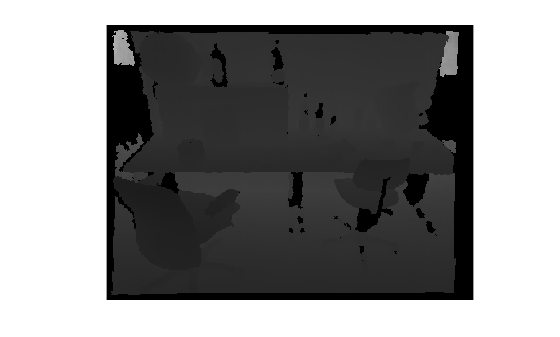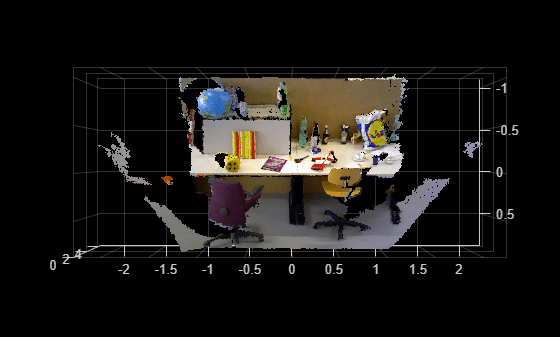# pcfromdepth

Convert depth image to point cloud

Since R2022b

## Syntax

``ptCloud = pcfromdepth(depthImage,depthScaleFactor,intrinsics)``
``ptCloud = pcfromdepth(___,Name=Value)``

## Description

example

````ptCloud = pcfromdepth(depthImage,depthScaleFactor,intrinsics)` converts a depth image using camera intrinsics, into a point cloud. The output point cloud is specified in 3-D world coordinates with its origin centered at the camera. The `depthScaleFactor`, normally provided by the RGB-D camera manufacturer specifies a division factor for the depth image.This function applies only for RGB-D cameras.```
````ptCloud = pcfromdepth(___,Name=Value)` specifies options using one or more name-value arguments in addition to any combination of arguments from previous syntaxes. For example, `pcfromdepth(depthImage,depthScaleFactor,intrinsics,DepthRange=[0,Inf])` sets the depth range to the 2-element vector value of `[0,Inf]`.```

## Examples

collapse all

Read and display the depth image taken from an RGB-D camera.

```depthImage = imread("sampleDepth.png"); imshow(depthImage)```Read and display the color image that corresponds to the depth image.

```colorImage = imread("sampleImage.png"); imshow("sampleImage.png")```Create a `cameraIntrinsics` object to store the camera intrinsics parameters. The focal length, principal point, and image size are in units of pixels. The maximum camera depth is in meters.

```focalLength = [535.4, 539.2]; principalPoint = [320.1, 247.6]; imageSize = size(depthImage,[1,2]); intrinsics = cameraIntrinsics(focalLength,principalPoint,imageSize); depthScaleFactor = 5e3; maxCameraDepth = 5;```

Convert depth map into world points, converting all points into point cloud.

```ptCloud = pcfromdepth(depthImage,depthScaleFactor, intrinsics, ... ColorImage=colorImage, ... DepthRange=[0 maxCameraDepth]);```

Display the resulting point cloud.

`pcshow(ptCloud, VerticalAxis="Y", VerticalAxisDir="Up", ViewPlane="YX");`Detect and extract ORB features from the color image.

`imagePoints = detectORBFeatures(im2gray(colorImage));`

Convert depth map into world points, converting only feature points into point cloud.

```ptCloud = pcfromdepth(depthImage,depthScaleFactor, intrinsics, ... ColorImage=colorImage, ... ImagePoints=imagePoints, ... DepthRange=[0 maxCameraDepth]);```

Display the resulting point cloud.

`pcshow(ptCloud, VerticalAxis="Y", VerticalAxisDir="Up", ViewPlane="YX");`## Input Arguments

collapse all

Depth image, specified as an M-by-N numeric matrix. Input images must be undistorted.

Depth correction factor, specified as a real scalar numeric type. This value is normally provided by the RGB-D camera manufacturer and specifies a division factor for the depth image input `depthImage`, which was taken with the camera. For example, for the world 3-D coordinates (X,Y,Z), where Z represents the depth, at any pixel coordinate (u,v), Z would be computed as:

`Z = depthImage[u,v]/depthScaleFactor`

Camera intrinsics, specified as a `cameraIntrinsics` object.

### Name-Value Arguments

Specify optional pairs of arguments as `Name1=Value1,...,NameN=ValueN`, where `Name` is the argument name and `Value` is the corresponding value. Name-value arguments must appear after other arguments, but the order of the pairs does not matter.

Before R2021a, use commas to separate each name and value, and enclose `Name` in quotes.

Example: `pcfromdepth(depthImage,depthScaleFactor,intrinsics,DepthRange=[0,Inf])` sets the depth range to the 2-element vector value of `[0,Inf]`.

Image points, specified as an M-by-2 matrix of [x y] coordinates, where M is the number of points, or by one of the feature point objects listed in Point Feature Types. Only points that are specified in the object are converted into a point cloud.

Color image, specified as an M-by-N-by-3 matrix of type numeric. The color image is associated with the depth image from the RGB-D camera.

Depth range of the RGB-D camera in world units, specified as a 1-by-2 vector. World units can be expressed as a measurement, such as meters. The range specifies the minimum and maximum depth range used to filter out invalid depth values after computing the point cloud.

Rectangular region of points to convert to a point cloud, specified as [x y width height]. [x y] specifies the upper-left corner of the rectangular region of interest.

## Output Arguments

collapse all

Point cloud created from depth image, returned as a `pointCloud` object. When you specify the `ImagePoints` name-value argument as an input, the function returns an unorganized point cloud. Otherwise, the function returns an organized point cloud.

 The TUM data set is provided by J. Sturm, N. Engelhard, F. Endres, W. Burgard and D. Cremers under the CC-BY-4.0 license license. All warranties and representations are disclaimed; see the license for details. MathWorks® modified the name of the RGB-D SLAM Dataset and Benchmark dataset to use in the example.